Question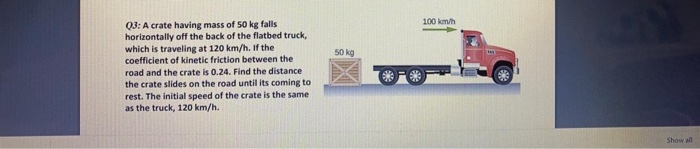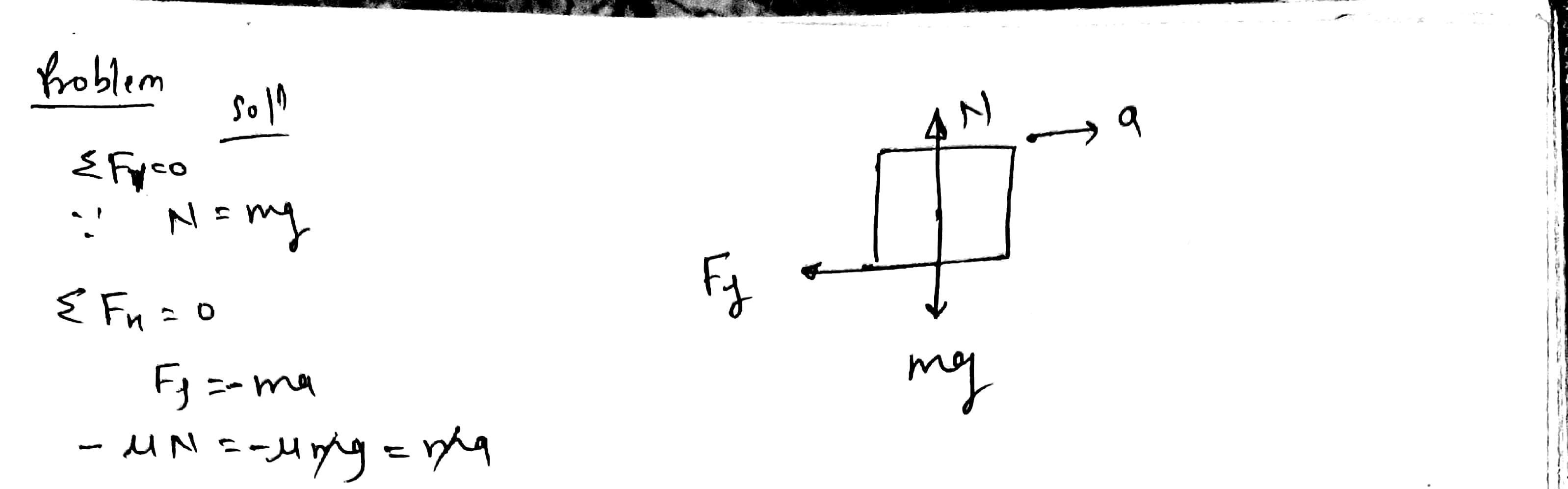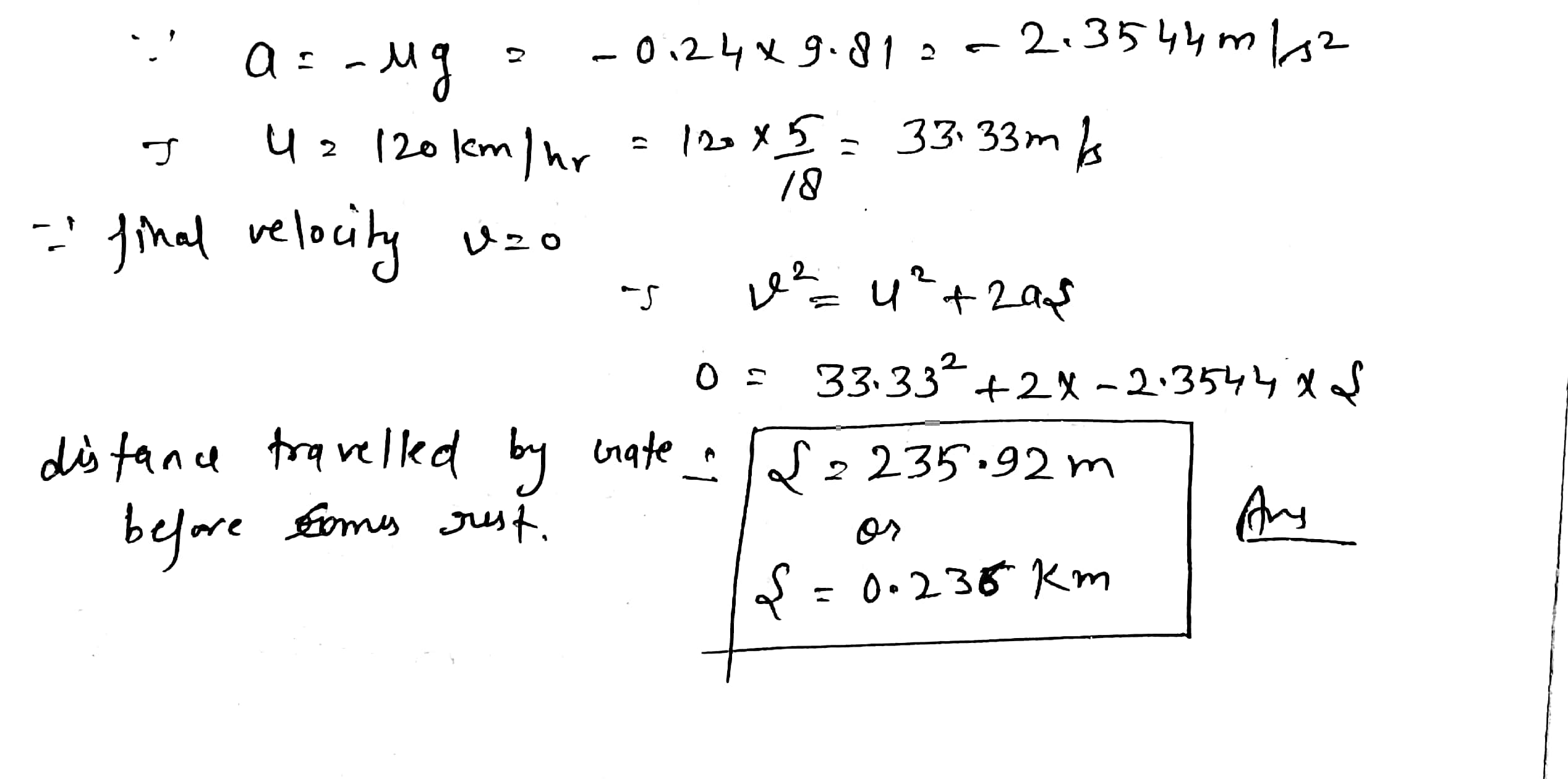#### Earn Coins

Coins can be redeemed for fabulous gifts.

Similar Homework Help Questions
• ### A crate having a mass 50.0 kg falls horizontally off the back of the flatbed truck,...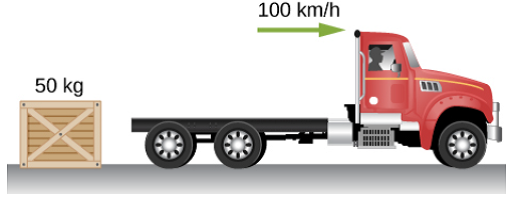A crate having a mass 50.0 kg falls horizontally off the back of the flatbed truck, which is traveling at 28 m/s. The crate slides 86.8 m on the road in coming to rest. The initial speed of the crate on the road is the same as that of the truck. (a). Draw and clearly label all of the forces acting on the crate as it slides on the road. (b). Draw the direction of the initial velocity for the...

• ### Problem 5.33 The coefficient of kinetic friction between a 50 kg crate and the warehouse floor...

Problem 5.33 The coefficient of kinetic friction between a 50 kg crate and the warehouse floor is 80 % of the corresponding coefficient of static friction. The crate falls off a forklift that is moving at4 m/s and then slides along the warehouse floor for a distance of 3.5 m before coming to rest. Part A What is the coefficient of static friction between the crate and the floor? Express your answer to one significant figure. #PLEASE SHOW WORK

• ### A crate of mass 29 kg is loaded onto the back of a flatbed truck

A crate of mass 29 kg is loaded onto the back of a flatbed truck. The coefficient of static friction between the box and the truck bed is 0.18. What is the maximum acceleration a (in m/s2) the truck can have without the crate sliding off?

• ### uestion 6 1.4 points Save Ans The crate, which has a mass of 100 kg, is...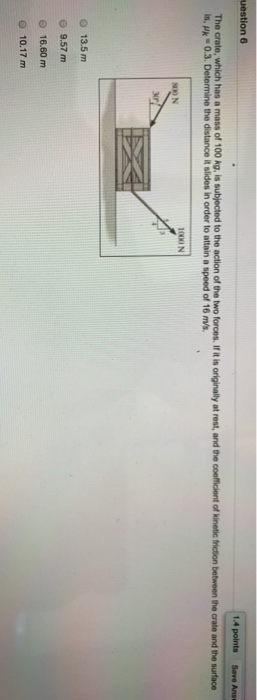uestion 6 1.4 points Save Ans The crate, which has a mass of 100 kg, is subjected to the action of the two forces. If it is originally at rest, and the coefficient of kinetic friction between the crate and the surface is, pk 0.3. Determine the distance it slides in order to attain a speed of 16 m/s 1000 N S0O N 30 O 13.5 m 9.57 m O 16.60 m O 10.17 m uestion 6 1.4 points Save...

• ### 7. show your work The crate, which has a mass of 100 kg, is subjected to...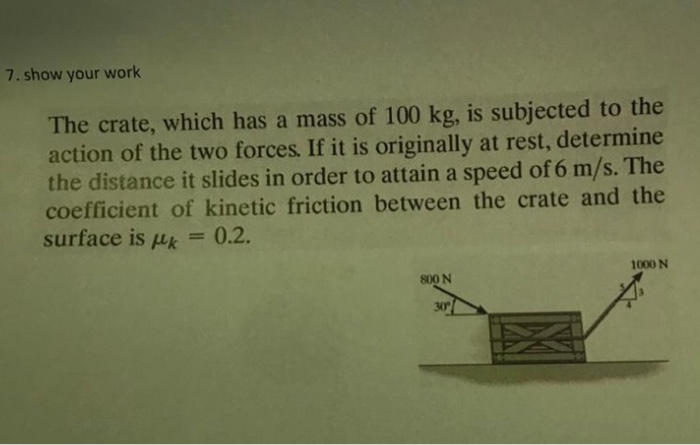7. show your work The crate, which has a mass of 100 kg, is subjected to the action of the two forces. If it is originally at rest, determine the distance it slides in order to attain a speed of 6 m/s. The coefficient of kinetic friction between the crate and the surface is uk 0.2. 1000 N 800 N 30

• ### A spring is mounted horizontally. A crate, which has a mass of 8.5 kg is pressed...

A spring is mounted horizontally. A crate, which has a mass of 8.5 kg is pressed against the spring with a force of 350N As a result the spring is compressed a distance of 82.0 cm The mass is then released and is allowed to slide along the horizontal surface which has friction. The coefficient of kinetic friction between the crate and the surface is 0.65 How much work will be done on the crate by the frictional force from...

• ### A box with a mass of 8.67 kg slides up a ramp inclined at an angle...

A box with a mass of 8.67 kg slides up a ramp inclined at an angle of 28.3° with the horizontal. The initial speed is 1.66 m/s and the coefficient of kinetic friction between the block and the ramp is 0.48. Determine the distance the block slides before coming to rest. m As shown in the figure below, a box of mass m = 35.0 kg is sliding along a horizontal frictionless surface at a speed vi = 5.55 m/s...

• ### QUESTION 3 The 1500-kg rear driving truck reaches a speed of 50 km/h from rest in...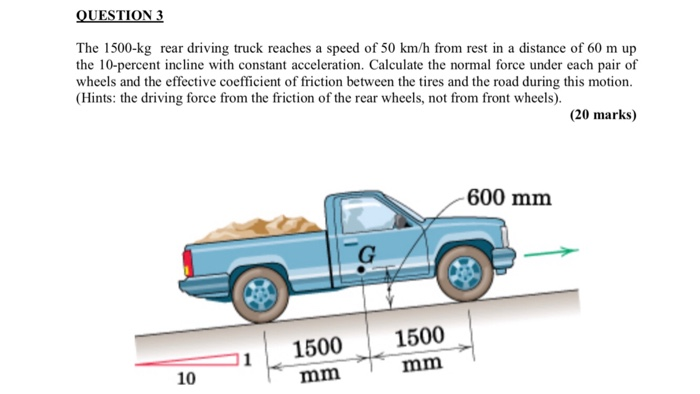QUESTION 3 The 1500-kg rear driving truck reaches a speed of 50 km/h from rest in a distance of 60 m up the 10-percent incline with constant acceleration. Calculate the normal force under each pair of wheels and the effective coefficient of friction between the tires and the road during this motion (Hints: the driving force from the friction of the rear wheels, not from front wheels). (20 marks) 600 mm 1500 mm 1500 mm 10 QUESTION 3 The 1500-kg...

• ### Question 2 (20 marks): A 1 kg ball A is traveling horizontally at 20 m/s when...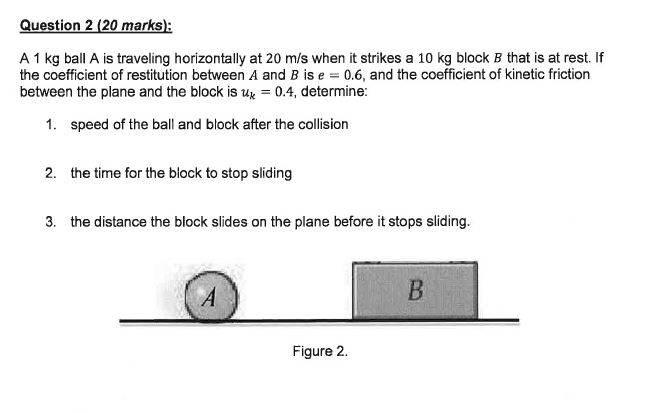Question 2 (20 marks): A 1 kg ball A is traveling horizontally at 20 m/s when it strikes a 10 kg block B that is at rest. If the coefficient of restitution between A and B is e = 0.6, and the coefficient of kinetic friction between the plane and the block is uy = 0.4, determine: 1. speed of the ball and block after the collision 2. the time for the block to stop sliding 3. the distance the...

• ### A softball having a mass of 0.30 kg is pitched horizontally at 120 km/h. By the...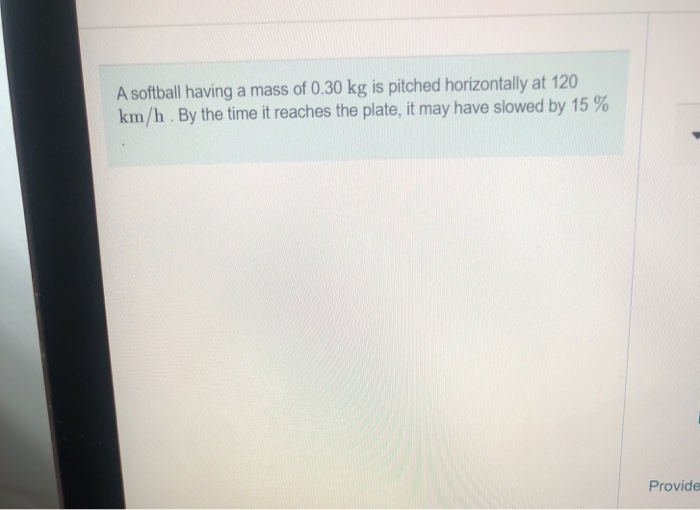A softball having a mass of 0.30 kg is pitched horizontally at 120 km/h. By the time it reaches the plate, it may have slowed by 15 % Provide Part A Neglecting gravity, estimate the average force of air resistance during a pitch, if the distance between the plate and the pitcher is about 20 m. Express your answer using two significant figures. | ΑΣφ ? N Submit Request Answer